GFG App
Open AppBrowser
Continue

# Solve Sudoku with Computer Vision and Constraint Satisfaction Algorithm

This article explains a program in python 2.7 to solve a Sudoku 9×9 of the Android application “Sudoku” of genina.com. To solve a sudoku of the Android application “Sudoku” of genina.com, a screenshot of the game is taken (a 720×1280 image is obtained), then the number found in each of the 81 squares is obtained using KNN algorithm, once each element is determined, the sudoku is solved using a constraint satisfaction algorithm with backtracking.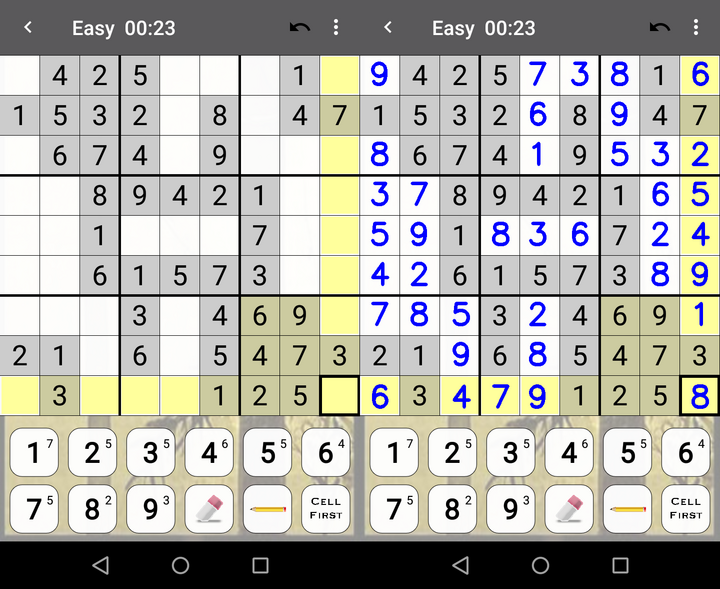On the left is our input: Screenshot that we are going to analyze. On the right is the solution.

How this work?
Step 1: Image Preprocessing
First step, Image Preprocessing: Extract each sudoku square individually and save them sequentially as photo # .png (where # goes from 0 to 80). Images of 80×75 pixels are obtained.
Code: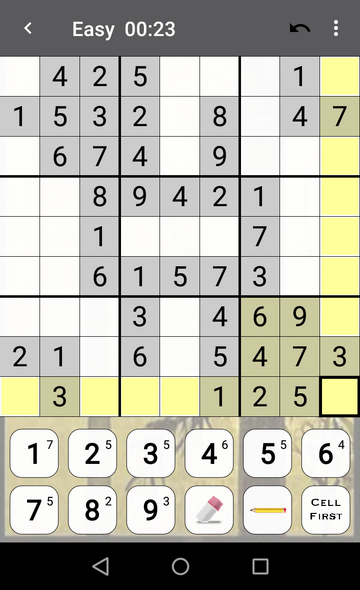Input: photo0.png. This is the photo that we are going to analyze.

Code:

## python

 `#/Preprocessing.py / import cv2` `import` `numpy as np` `import` `Functions`   `# Relative path` `path ``=``".``/``Screenshots``/``"`   `# Image to analyze` `number ``=` `input``("Enter image number: ")` `globalPath ``=` `path``+``"photo"``+``str``(number)``+``".png"` `image ``=` `cv2.imread(globalPath)`   `# Save the name of the image to analyze after in Main.py` `file` `=` `open``("image.txt", "w")` `file``.write(globalPath)` `file``.close()`   `# MAIN` `if` `__name__ ``=``=` `'__main__'``:    ` `    `  `    ``# PREPROCESSING -> Crop the edges, ads and all ` `    ``# the images outside the sudoku board` `    ``image ``=` `Functions.cropImage(image, ``218``)` `    ``image ``=` `Functions.rotateImage(image, ``180``)` `    ``image ``=` `Functions.cropImage(image, ``348``)` `    ``image ``=` `Functions.rotateImage(image, ``180``)` `    `  `    ``# Crop each box in the sudoku board` `    ``cont ``=` `0` `    ``w ``=` `0` `    ``for` `j ``in` `range``(``9``):` `        ``h ``=` `0`   `        ``for` `i ``in` `range``(``9``):`   `            ``nombre ``=` `"image"``+` `str``(cont) ``+` `".png"` `            ``image1 ``=` `Functions.cropBox(image, w, h, ``75``, ``80``)`   `            ``# Save the image` `            ``Functions.saveImage(image1, nombre)` `            ``h ``=` `h ``+` `80` `            ``cont ``=` `cont ``+` `1`   `        ``# Position of the pixel where start the image` `        ``w ``=` `80``*``(j ``+` `1``)`

Code: create a library with functions for only preprocessing and image transformation called “Functions”.

## python

 `#/Functions.py / import cv2` `import` `numpy as np`   `# Function to rotate the image` `def` `rotateImage(image, angle):` `     ``image_center ``=` `tuple``(np.array(image.shape[``1``::``-``1``]) ``/` `2``)` `     ``rot_mat ``=` `cv2.getRotationMatrix2D(image_center, angle, ``1.0``)` `     ``result ``=` `cv2.warpAffine(image, rot_mat, image.shape[``1``::``-``1``], flags ``=` `cv2.INTER_LINEAR)` `     ``return` `result` ` `  `# Function to crop top border in the image` `def` `cropImage(image, x):`   `    ``# x determine how far to cut the image` `    ``# fileb determines with what name we are going to save the image` `    ``# Determine image dimensions` `    ``height, width, channels ``=` `image.shape` `    ``crop_img ``=` `image[x:height, ``0``:width]` `    ``return` `crop_img`   `# Function to crop every box (there are 81 boxes in total) ` `def` `cropBox(image, x, y, h, w):` `    ``# Each side of the square / box has a side of length 10` `    ``crop_img ``=` `image[x:(x ``+` `h), y:(y ``+` `w)]` `    ``return` `crop_img`   `# Function to save the image ` `def` `saveImage(image, fileb):` `    ``new_path ``=` `".``/``Images``/``"` `    ``cv2.imwrite(new_path ``+` `fileb, image)` `    ``cv2.waitKey(``0``)` `    ``cv2.destroyAllWindows()`   `# Function to crop all borders of each box` `def` `cropBorder(image):`   `    ``# Determine image dimensions` `    ``height, width, channels ``=` `image.shape` `    ``crop_img ``=` `image[``12``:height``-``12``, ``12``:width``-``12``]` `    ``return` `crop_img`

Step 2: Image Transformation
Cut out the borders of each box, in case there is any black border that can be inferred in our analysis.Each image has 56×51 pixels.
Code:

## python

 `#/Transformation.py / import cv2` `import` `numpy as np` `import` `Functions`   `# Relative path ` `path ``=``".``/``Images``/``"`   `if` `__name__ ``=``=` `'__main__'``:` `    `  `    ``for` `x ``in` `range``(``81``):`   `        ``# Image to analyze` `        ``nameImage ``=` `"image" ``+` `str``(x) ``+` `".png"` `        ``image ``=` `cv2.imread(path ``+` `nameImage)` `        ``image ``=` `Functions.cropBorder(image)` `        ``Functions.saveImage(image, nameImage)`

Step 3: KNN Classification
Analyze what number is in the box. In this case, Canny algorithm is used to determine if there is a number or it is an empty box. Then through the KNN algorithm it is determined which number is in the box. For the extraction of characteristics, the moments of Hu: 1 and 2, Gaussian filter for filtering and unsupervised thresholding were used.
Code:

## python

 `#/Preprocessing.py / import cv2` `import` `numpy as np` `import` `Functions`   `# Relative path` `path ``=``".``/``Screenshots``/``"`   `# Image to analyze` `number ``=` `input``("Enter image number: ")` `globalPath ``=` `path``+``"photo"``+``str``(number)``+``".png"` `image ``=` `cv2.imread(globalPath)`   `# Save the name of the image to analyze after in Main.py` `file` `=` `open``("image.txt", "w")` `file``.write(globalPath)` `file``.close()`   `# MAIN` `if` `__name__ ``=``=` `'__main__'``:    ` `    `  `    ``# PREPROCESSING -> Crop the edges, ads and all ` `    ``# the images outside the sudoku board` `    ``image ``=` `Functions.cropImage(image, ``218``)` `    ``image ``=` `Functions.rotateImage(image, ``180``)` `    ``image ``=` `Functions.cropImage(image, ``348``)` `    ``image ``=` `Functions.rotateImage(image, ``180``)` `    `  `    ``# Crop each box in the sudoku board` `    ``cont ``=` `0` `    ``w ``=` `0` `    ``for` `j ``in` `range``(``9``):` `        ``h ``=` `0`   `        ``for` `i ``in` `range``(``9``):`   `            ``nombre ``=` `"image"``+` `str``(cont) ``+` `".png"` `            ``image1 ``=` `Functions.cropBox(image, w, h, ``75``, ``80``)`   `            ``# Save the image` `            ``Functions.saveImage(image1, nombre)` `            ``h ``=` `h ``+` `80` `            ``cont ``=` `cont ``+` `1`   `        ``# Position of the pixel where start the image` `        ``w ``=` `80``*``(j ``+` `1``)`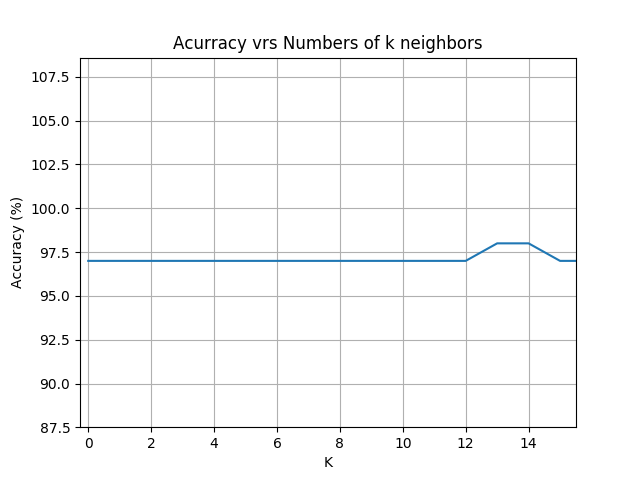Show performance KNN algorithm

Vector.txt contains all the elements extracted from the screenshot (where the boxes were scrolled from left to right, from top to bottom). In this project, the performance of the KNN algorithm presented a 97% accuracy with respect to all the images analyzed in the Test. In case of any error in the recognition of the numbers there is the option to manually change a prediction of the box in the vector.txt.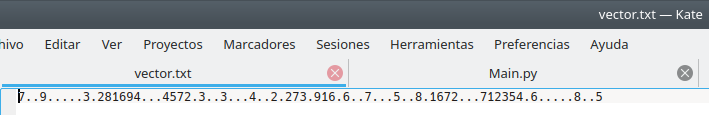Result of the recognition of all the digits of the sudoku grid of the image photo0.jpg

Step4: Now solve the sudoku!
A restriction satisfaction algorithm with backtracking is presented to solve the sudoku.
Code:

## python

 `#/Solver.py / import numpy as np`   `# Dictionary with grid numbers` `def` `solverGrid(grid):` `    `  `    ``values ``=` `valuesGrid(grid)` `    ``return` `searchValues(values)`   `# Exchange of items` `def` `exchangeValues(A, B):` `    `  `    ``return` `[a ``+` `b ``for` `a ``in` `A ``for` `b ``in` `B]`   `# Define initial values` `def` `initialValues(grid):` `    ``return` `dict``(``zip``(sections, grid))`   `# Define values in the grid` `def` `valuesGrid(grid):` `    ``numbers ``=` `[]`   `    ``for` `c ``in` `grid:` `        ``if` `c ``=``=` `'.'``:` `            ``numbers.append(``'123456789'``)` `        ``elif` `c ``in` `'123456789'``:` `            ``numbers.append(c)`   `    ``return` `dict``(``zip``(sections, numbers))`   `# Delete the values that are already inside the grid` `def` `eliminateValues(numbers):` `    `  `    ``solved_values ``=` `[box ``for` `box ``in` `numbers.keys() ``if` `len``(numbers[box]) ``=``=` `1``]` `    ``for` `box ``in` `solved_values:` `        ``digit ``=` `numbers[box]` `        ``for` `vecino ``in` `neighbors[box]:` `            ``numbers[vecino] ``=` `numbers[vecino].replace(digit, '')` `    ``return` `numbers`   `def` `onlyOption(numbers):` `    ``for` `unit ``in` `unitlist:` `        ``for` `digit ``in` `'123456789'``:` `            ``dplaces ``=` `[box ``for` `box ``in` `unit ``if` `digit ``in` `numbers[box]]` `            ``if` `len``(dplaces) ``=``=` `1``:` `                ``numbers[dplaces[``0``]] ``=` `digit` `    ``return` `numbers`   `def` `reduceSudoku(numbers):` `    ``stalled ``=` `False` `    ``while` `not` `stalled:` `        ``# Check how many boxes have a determined value` `        ``solved_values_before ``=` `len``([box ``for` `box ``in` `numbers.keys() ``if` `len``(numbers[box]) ``=``=` `1``])`   `        ``# Set the Eliminate Strategy` `        ``numbers ``=` `eliminateValues(numbers)`   `        ``# Use the Only Choice Strategy` `        ``numbers ``=` `onlyOption(numbers)`   `        ``# Check how many boxes have a determined value, to compare` `        ``solved_values_after ``=` `len``([box ``for` `box ``in` `numbers.keys() ``if` `len``(numbers[box]) ``=``=` `1``])`   `        ``# If no new values were added, stop the loop.` `        ``stalled ``=` `solved_values_before ``=``=` `solved_values_after`   `        ``# Sanity check, return False if there is a box with zero available values:` `        ``if` `len``([box ``for` `box ``in` `numbers.keys() ``if` `len``(numbers[box]) ``=``=` `0``]):` `            ``return` `False`   `    ``return` `numbers`   `def` `searchValues(numbers):`   `    ``numbers ``=` `reduceSudoku(numbers)`   `    ``if` `numbers ``is` `False``:` `        ``return` `False`    `## Failure` `    ``if` `all``(``len``(numbers[s]) ``=``=` `1` `for` `s ``in` `sections): ` `        ``return` `numbers  ``## Ok` `    `  `    ``# Choose one of the unfilled boxes` `    ``unfilled_squares ``=` `[(``len``(numbers[s]), s) ``for` `s ``in` `sections ``if` `len``(numbers[s]) > ``1``]` `    ``n, s ``=` `min``(unfilled_squares)` `    `  `    ``# Solve the next boxes` `    ``for` `value ``in` `numbers[s]:` `        ``nova_sudoku ``=` `numbers.copy()` `        ``nova_sudoku[s] ``=` `value` `        ``attempt ``=` `searchValues(nova_sudoku)` `        ``if` `attempt:` `            ``return` `attempt`   `# Define values` `rows ``=` `'ABCDEFGHI'` `columns ``=` `'123456789'`   `sections ``=` `exchangeValues(rows, columns)` `rowsUnit ``=` `[exchangeValues(r, columns) ``for` `r ``in` `rows]` `columnUnits ``=` `[exchangeValues(rows, c) ``for` `c ``in` `columns]` `boxUnits ``=` `[exchangeValues(rs, cs) ``for` `rs ``in` `(``'ABC'``, ``'DEF'``, ``'GHI'``) ``for` `cs ``in` `(``'123'``, ``'456'``, ``'789'``)]`   `unitlist ``=` `rowsUnit ``+` `columnUnits ``+` `boxUnits`   `units ``=` `dict``((s, [u ``for` `u ``in` `unitlist ``if` `s ``in` `u]) ``for` `s ``in` `sections)` `neighbors ``=` `dict``((s, ``set``(``sum``(units[s], []))``-``set``([s])) ``for` `s ``in` `sections)`   `# MAIN` `if` `__name__ ``=``=` `'__main__'``:` `    `  `    ``# With file manager to read the file vector.txt ` `    ``# that has all the values of the screenshot` `    ``file` `=` `open``("vector.txt", "r")` `    ``lines ``=` `file``.read()` `    ``file``.close() `   `    ``# Access the dictionary` `    ``a ``=` `solverGrid(lines)` `    ``b ``=` `sorted``(a.items())` `    `  `    ``# Save the dictionary solution` `    ``np.save(``'Solution'``, b) `

Step 5: Interface
Improves the way the solution is displayed compared to the original screenshot.
Code:

## python

 `#/Interface.py / ` `import` `numpy as np` `import` `matplotlib.pyplot as plt` `import` `cv2`   `# Read dictionary from Solution.npy` `readDictionary ``=` `np.load(``'Solution.npy'``)` `values ``=` `(readDictionary[:, ``1``])`   `# Read vector.txt` `file` `=` `open``("vector.txt", "r")` `lines ``=` `file``.read()` `file``.close()`   `# Read the path of the image the we want to analyze` `fileTxt ``=` `open``("image.txt", "r")` `pathGlobal ``=` `fileTxt.read()` `fileTxt.close()`   `# Obtain the coordinates to be able to ` `# locate them in the image` `row ``=` `["A", "B", "C", "D", "E", "F", "G", "H", "I"]` `column ``=` `["``1``", "``2``", "``3``", "``4``", "``5``", "``6``", "``7``", "``8``", "``9``"]`   `# Assign the coordinates of each number within the image plane` `def` `coordinate():` `    ``positionx ``=` `list``()` `    ``positiony ``=` `list``()` `    `  `    ``for` `k ``in` `range``(``9``):` `        ``for` `i ``in` `range``(``9``):` `        `  `            ``if` `(row[k] ``=``=` `"A"): y ``=` `270` `            ``elif` `(row[k] ``=``=` `"B"): y ``=` `350` `            ``elif` `(row[k] ``=``=` `"C"): y ``=` `430` `            ``elif` `(row[k] ``=``=` `"D"): y ``=` `510` `            ``elif` `(row[k] ``=``=` `"E"): y ``=` `590` `            ``elif` `(row[k] ``=``=` `"F"): y ``=` `670` `            ``elif` `(row[k] ``=``=` `"G"): y ``=` `750` `            ``elif` `(row[k] ``=``=` `"H"): y ``=` `830` `            ``elif` `(row[k] ``=``=` `"I"): y ``=` `915` `        `  `            ``if` `(column[i] ``=``=` `"``1``"): x ``=` `19` `            ``elif` `(column[i] ``=``=` `"``2``"): x ``=` `98` `            ``elif` `(column[i] ``=``=` `"``3``"): x ``=` `182` `            ``elif` `(column[i] ``=``=` `"``4``"): x ``=` `261` `            ``elif` `(column[i] ``=``=` `"``5``"): x ``=` `335` `            ``elif` `(column[i] ``=``=` `"``6``"): x ``=` `419` `            ``elif` `(column[i] ``=``=` `"``7``"): x ``=` `499` `            ``elif` `(column[i] ``=``=` `"``8``"): x ``=` `580` `            ``elif` `(column[i] ``=``=` `"``9``"): x ``=` `660`   `            ``positionx.append(x)` `            ``positiony.append(y)` `        `  `    ``return` `(positionx, positiony)        `   `# Function to write value in each box in the image` `def` `writeValue(image, valor, x, y):` `        `  `    ``font ``=` `cv2.FONT_HERSHEY_SIMPLEX` `    ``text ``=` `str``(valor)` `    `  `    ``# Write text in the image` `    ``cv2.putText(image, text, (x, y), font, ``2``, (``255``, ``0``, ``0``), ``5``)` `    ``# cv2.putText(image, text, (coordinates), size font, (color RGB), thickness)` `    `  `    ``return` `image`   `# Load image` `image ``=` `cv2.imread(pathGlobal)` `image2 ``=` `image.copy()`   `# Load coordinates` `positionx, positiony ``=` `coordinate()`   `for` `i ``in` `range``(``81``):` `    ``if` `(lines[i] ``=``=` `"."):` `        ``image ``=` `writeValue(image, values[i], positionx[i], positiony[i])`   `# Concatenate images horizontally` `image ``=` `np.concatenate((image2, image), axis ``=` `1``)`   `# Show image concatenation   ` `plt.imshow(image)` `plt.axis("off")` `plt.show()`   `# Save image` `cv2.imwrite(".``/``Interface ``/` `example.png", image)`

Output: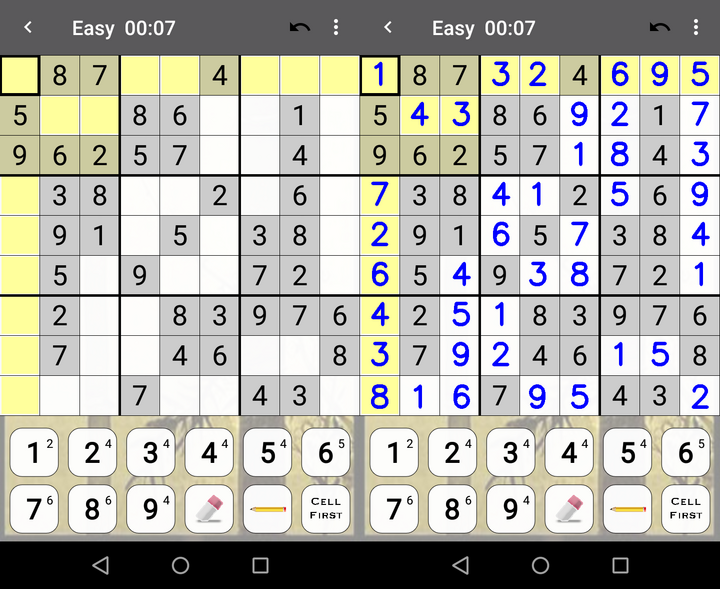Results for photo1.png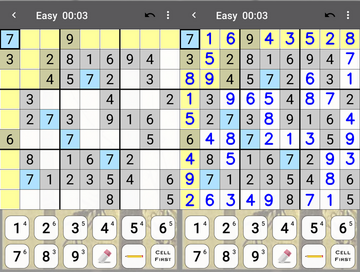Results for photo2.png

All the images for the training of the KNN algorithm and the screenshots of example can be found in given repository

My Personal Notes arrow_drop_up